# I.E. Irodov Solutions on Hydrodynamics

Students can find the solutions of the Problems In General Physics I.E. Irodov - Hydrodynamics on this page. The branch of physics that deals with the motion of fluids is called hydrodynamics. This is an important topic in the physical fundamentals of mechanics. The main topics include Eulerian equation, Bernoulli's equation, Reynolds number, Poiseuille's law, Stokes' law, etc. Students can expect one question from this section for different entrance exams and board exams.

Practising these solutions will help students to improve their speed and accuracy and thus achieve better results for the JEE Main and other exams.

### I.E. Irodov Solutions on Hydrodynamics

1. Ideal fluid flows along a flat tube of constant cross-section, located in a horizontal plane and bent as shown in Fig. 1.80 (top view). The flow is steady. Are the pressures and velocities of the fluid equal at points 1and 2? What is the shape of the streamlines?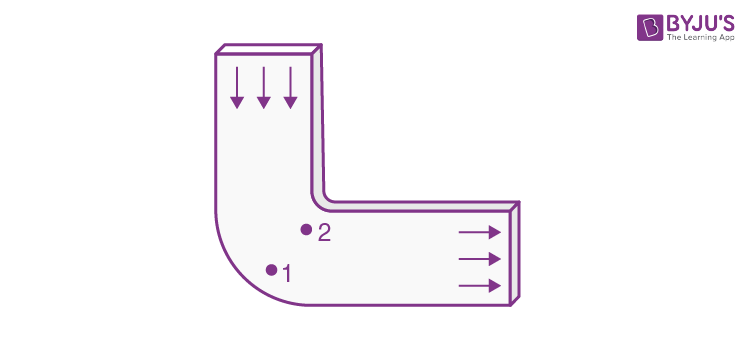Solution:

1. Between 1 and 2 fluid particles are in nearly circular motion and therefore have centripetal acceleration. The force for this acceleration, like for any other situation in an ideal fluid, can only come from the pressure variation along the line joining 1 and 2. This requires that pressure at 1 should be greater than the pressure at 2 i.e.

P1 > P2

so that the fluid particles can have the required acceleration. If there is no turbulence, the motion can be taken as irrotational. Then by considering

\oint \vec{v}.d\vec{l}=0

Along the circuit shown we infer that

v2 > v1

(The portion of the circuit near 1 and 2 are streamlines while the other two arms are at right angle to streamlines)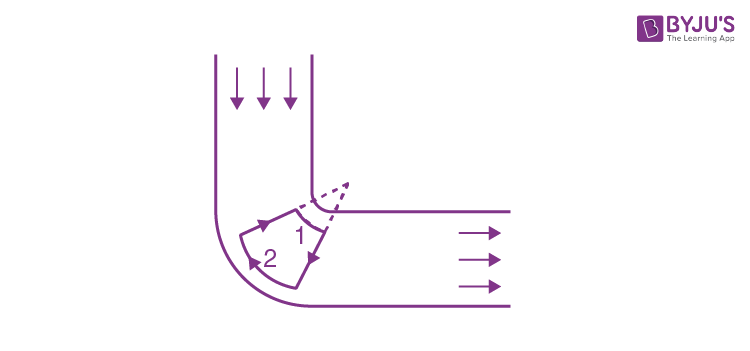In an incompressible liquid we also have div \vec{v}=0

By electrostatic analogy, we then find that the density of streamlines is proportional to the velocity at that point.

2. Two manometric tubes are mounted on a horizontal pipe of varying cross-section at the sections S1 and S2 (Fig. 1.81). Find the volume of water flowing across the pipe's section per unit time if the difference in water columns is equal to ∆h.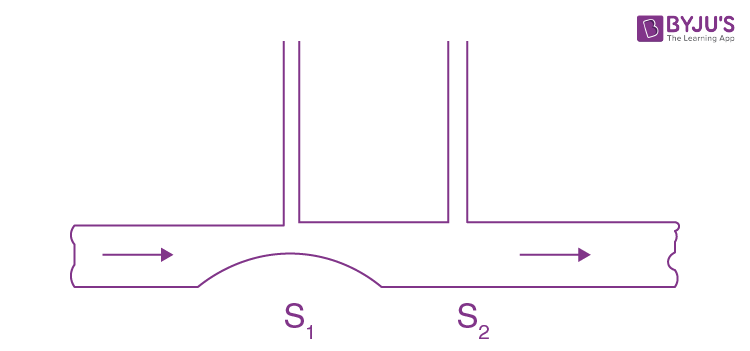Solution:

1. From the conservation of mass

v1S1 = v2S2 (1)

But S1 < S2 as shown in the figure of the problem, therefore

v1>v2

As every streamline is horizontal 1 and 2, Bernoulli’s theorem becomes

p + ½ ρv2 = constant, which gives

p1<p2 as v1>v2

As the difference in height of the water column is ∆h, therefore

p2-p1 = ρg∆h (2)

From Bernoulli’s theorem between points 1 and 2 of a streamline

p1 + ½ ρv12 = p2 + ½ ρv22

Or p2 - p1 = ½ ρ(v12 - v22)

Or ρg∆h = ½ ρ(v12 - v22) (3) (using Eq. 2)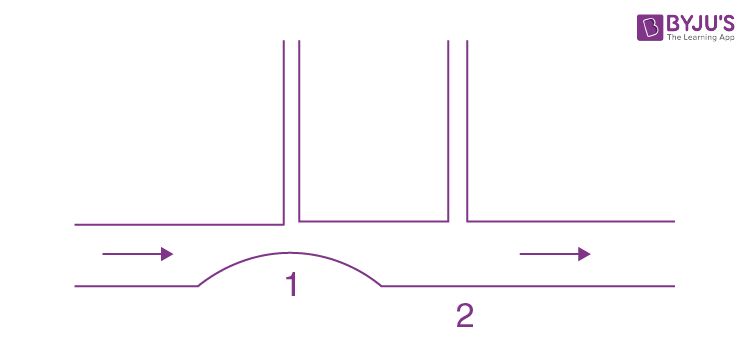Using (1) in (3), we get

v1 = S2√(2g∆h/(S22 - S12))

Hence the sought volume of water flowing per sec

Q = v1S1 = S1S2√(2g∆h/(S22 - S12))

3. A Pitot tube (Fig. 1.82) is mounted along the axis of a gas pipeline whose cross-sectional area is equal to S. Assuming the viscosity to be negligible, find the volume of gas flowing across the section of the pipe per unit time, if the difference in the liquid columns is equal to Δh, and the densities of the liquid and the gas are ρ0 and ρ respectively.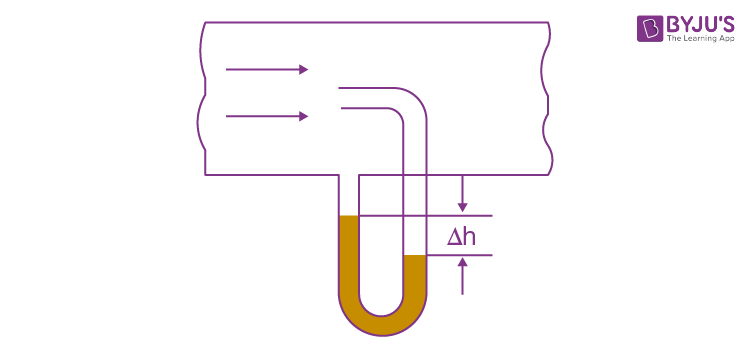Solution:

1. Applying Bernoulli’s theorem for the point A and B,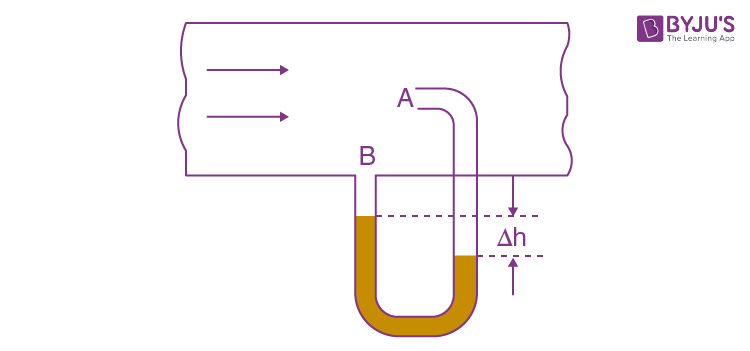pA = pB + ½ρv2 as vA = 0

Or ½ ρv2 = pA - pB = ∆hρ0g

So v = √(2∆hρ0g/ρ)

Thus, rate of flow of gas, Q = Sv

= S √(2∆hρ0g/ρ)

The gas flows over the tube past it at B. But at A the gas becomes stationary as the gas will move into the tube which already contains gas. On applying Bernoulli’s theorem we should remember that

p/ρ + ½ v2 + gz is constant along a streamline. In the present case, we are really applying Bernoulli's theorem somewhat indirectly. The streamline at A is not the streamline at B. Nevertheless the result is correct. To be convinced of this, we need only apply Bernoulli theorem to the streamline that goes through A by comparing the situation at A with that above B on the same level. In steady conditions, this agrees with the result derived because there cannot be a transverse pressure differential.

4. A wide vessel with a small hole in the bottom is filled with water and kerosene. Neglecting the viscosity, find the velocity of the water flow, if the thickness of the water layer is equal to h1 = 30 cm and that of the kerosene layer to h2 = 20 cm.

Solution:

1. Since the density of water is greater than that of kerosene oil, it will collect at the bottom. Now, pressure due to water level equals h1ρ1g and pressure due to kerosene oil level equals h2ρ2g. So, net pressure becomes h1ρ1g + h2ρ2g. From Bernoulli’s theorem, this pressure energy will be converted into kinetic energy while flowing through the whole A.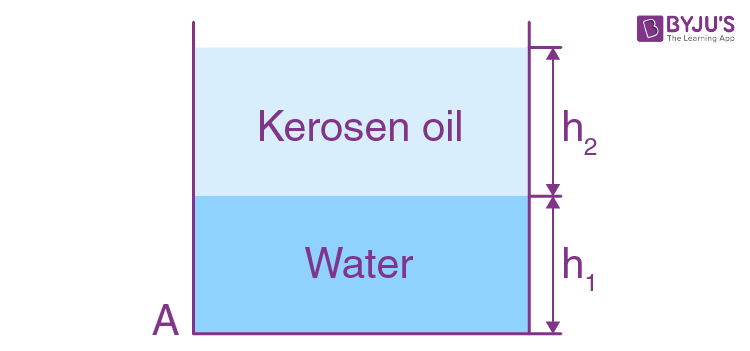i.e h1ρ1g + h2ρ2g = ½ ρ1v2

Hence v = √(2(h1 + h2 ρ21)g)

= 3 m/s

5. A wide cylindrical vessel 50 cm in height is filled with water and rests on a table. Assuming the viscosity to be negligible, find at what height from the bottom of the vessel a small hole should be perforated for the water jet coming out of it to hit the surface of the table at the maximum distance lmax from the vessel. Find lmax.

Solution:

1. Let, H be the total height of the water column and the hole is made at a height h from the bottom.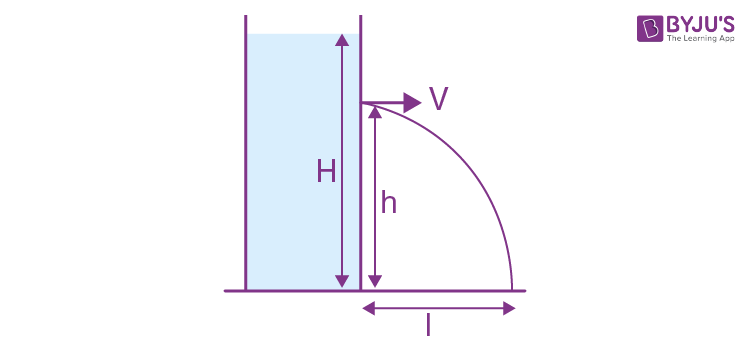Then from Bernoulli’s theorem

½ ρv2 = (H - h)ρg

Or v = √((H - h)2g), which is directed horizontally.

For the horizontal range, l = vt

= √(2g(H - h)) .√(2h/g)

= 2√(Hh - h2)

Now, for maximum l, d(Hh - h2/dh = 0 which yields h = H/2 = 25 cm

6. A bent tube is lowered into a water stream as shown in Fig. 1.83. The velocity of the stream relative to the tube is equal to v = 2.5 m/s. The closed upper end of the tube located at the height h0 = 12cm has a small orifice. To what height h will the water jet spurt?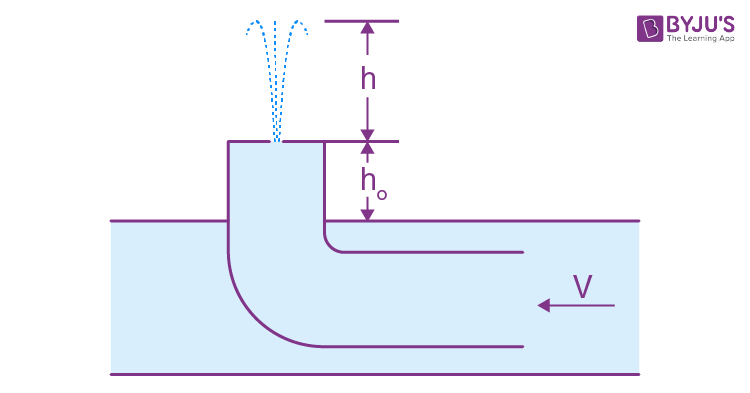Solution:

1. Let the velocity of the water jet, near the orifice, be v’, then applying Bernoullis theorem,

½ ρv2 = h0ρg + ½ ρv2

Or v’ = √(v2 - 2gh0) (1)

Here the pressure term on both sides is the same and equal to atmospheric pressure. Now, if it rises upto a height h, then at this height, whole of its kinetic energy will be converted into potential energy. So,

½ ρv’2 = ρgh

Or h = v’2/2g

= v2/2g - h0

= 20 cm (using Eq. (1))

7. The horizontal bottom of a wide vessel with an ideal fluid has a round orifice of radius R1 over which a round closed cylinder is mounted, whose radius R2 > R1 ( Fig. 1.84). The clearance between the cylinder and the bottom of the vessel is very small, the fluid density is ρ. Find the static pressure of the fluid in the clearance as a function of the distance r from the axis of the orifice (and the cylinder), if the height of the fluid is equal to h.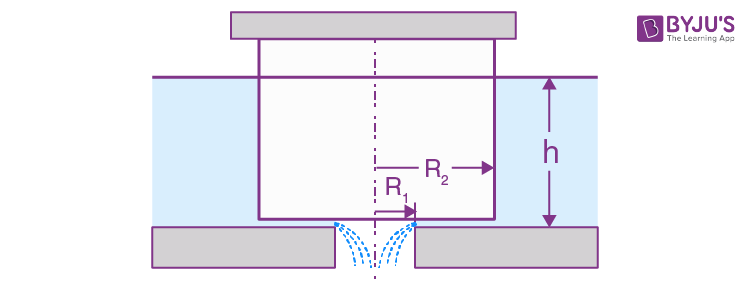Solution:

1. Water flows through the small clearance into the orifice. Let d be the clearance. Then from the equation of continuity

(2πR1d)v1 = (2πrd)v = (2πR2d)v2

Or v1R1 = vr = v2R2 (1)

where v1, v2, and v are respectively the inward radial velocities of the fluid at 1, 2, and 3.

Now by Bernoulli’s theorem just before 2 and just after it in the clearance

p0 + hρg = p2 + ½ ρv22 (2)

Applying the same theorem at 3 and 1 we find that this also equals

p + ½ ρv2 = p0 + ½ ρv12 (3)

(Since the pressure in the orifice is p0)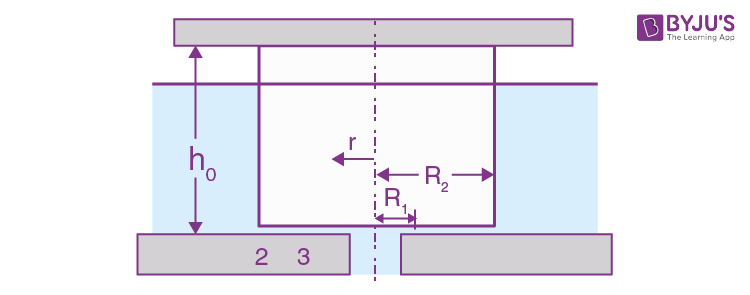From Eqs. (2) and (3) we also hence

v1 = √(2gh) (4)

And p = p0 + ½ ρv12 (1 - (v/v1)2)

= p0 + hρg (1 - (R1/r)2) (using (1) and (4))

8. What work should be done in order to squeeze all water from a horizontally located cylinder (Fig. 1.85) during the time t by means of a constant force acting on the piston? The volume of water in the cylinder is equal to V, the cross-sectional area of the orifice to s, with s being considerably less than the piston area. The friction and viscosity are negligibly small.Solution:

1.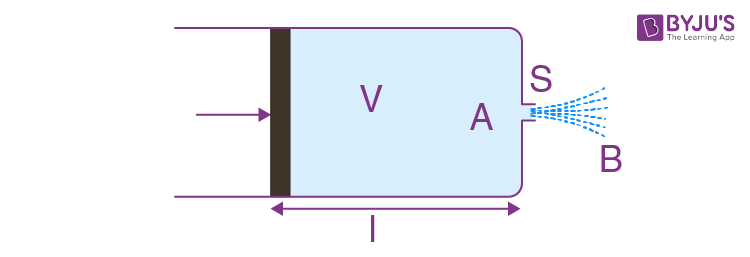Let the force acting on the piston be F and the length of the cylinder bel.

Then, work done = FI (1)

Applying Bernoulli's theorem for points A and B.

p = ½ ρv2

where ρ is density and v is the velocity at point B.

Now, force on the piston,

F = pA = ½ ρv2A (2)

where A is the cross-section area of the piston. Also, discharge through the orifice during time interval t = Svt and this is equal to the volume of the cylinder, i.e

V = Svt or v = V/St (3)

From Eq. (1), (2) and (3) work done

= ½ ρv2Al

= ½ ρAV2l/(St)2

= ½ ρV3/S3t2 (as Al = V)

9. A cylindrical vessel of height h and base area S is filled with water. An orifice of area s << S is opened in the bottom of the vessel. Neglecting the viscosity of water, determine how soon all the water will pour out of the vessel.

Solution:

1. Let at any moment of time, water level in the vessel be H, then speed of flow of water through the orifice, at that moment will be

v = √(2gH) (1)

In the time interval dt, the volume of water ejected through orifice,

dV = sv dt (2)

On the other hand, the volume of water in the vessel at time t equals

V = SH

Differentiating (3) w.r.t.time,

dV/dt = S dH/dt

Or dV = S dH (4)

Eqs. (2) and (4)

S dH = sv dt

Or dt = S dH/s√(2gH) from (2)

Integrating, \int_{0}^{t}dt=\frac{S}{s\sqrt{2h}}\int_{h}^{0}\frac{dh}{\sqrt{H}}

Thus t = (S/s) √(2h/g)

10. A horizontally oriented tube AB of length l rotates with a constant angular velocity ω about a stationary vertical axis OO' passing through the end A (Fig. 1.86). The tube is filled with an ideal fluid. The end A of the tube is open, the closed end B has a very small orifice. Find the velocity of the fluid relative to the tube as a function of the column height h.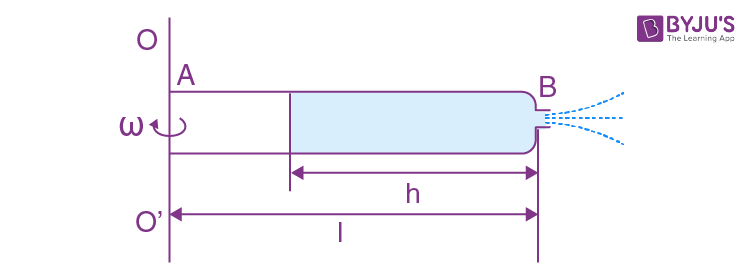Solution:

1. In a rotating frame (with constant angular velocity) the Eulerian equation is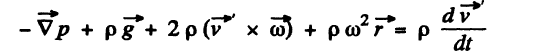In the frame of rotating tube the liquid in the column is practically static because the orifice is sufficiently small.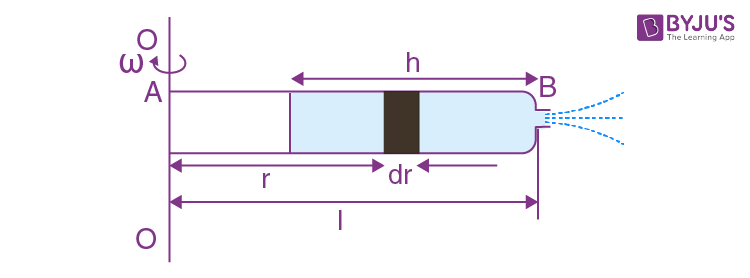Thus the Eulerian Eq. in projection form along vector r (which is the position vector of an arbitrary liquid element of length dr relative to the rotation axis) reduces to

-dp/dr + ρω2r = 0

Or dp = ρω2r dr

So \int_{p_{0}}^{p}dp=\rho \omega ^{2}\int_{l-h}^{r}r\: dr

Thus p(r) = p0 + (ρω2/2)(r2 - (l - h)2) (1)

Hence the pressure at the end B just before the orifice i.e.

p(l) = p0 + (ρω2/2)(2lh- h)2 (2)

Then applying Bernoulli's theorem at the orifice for the points just inside and outside of the end B.

p0 + ½ ρω2 (2lh - h2) = p0 + ½ ρv2 (where v is the sought velocity)

So v = ωh√(2l/h - 1)

11. Demonstrate that in the case of a steady flow of an ideal fluid Eq. (1.7 a) turns into Bernoulli equation.

Solution:

1. The Euler’s equation

\rho \frac{d\vec{v}}{dt}=\vec{f}-\vec{\triangledown} p=-\vec{\triangledown}(p+\rho gz)

where z is vertically upwards.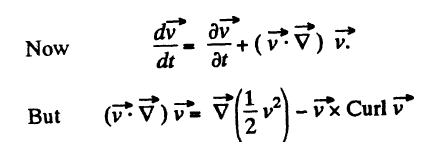we consider the steady flow of an incompressible fluid then p = constant and as the motion is irrotational curl \vec{v}=0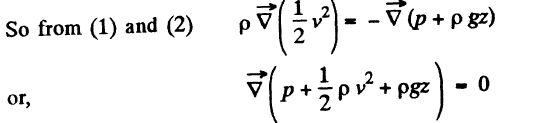Hence p + ½ ρv2 + ρgz = constant.

12. On the opposite sides of a wide vertical vessel filled with water two identical holes are opened, each having the cross sectional area S = 0.50 cm2. The height difference between them is equal to ∆h = 51 cm. Find the resultant force of reaction of the water flowing out of the vessel.

Solution:

1. Let the velocity of water, flowing through A be vA and that through B be vB , then discharging rate through A = QA = S vA and similarly through B = SvB.

Now, force of reaction at A,

FA = ρQAvA = ρSvB2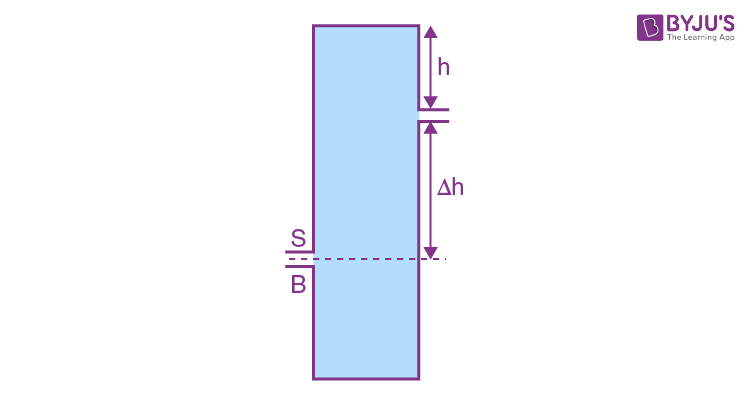Applying Bernoulli’s theorem to the liquid flowing out of A we get

ρ0 + ρgh = ρ0 + ½ ρvA2

And similarly at B

ρ0 + ρg(h+∆h) = ρ0 + ½ ρvB2

Hence (vB2 - vA2)ρ/2 = ∆hρg

Thus F = 2ρgS∆h = 0.50 N

13. The side wall of a wide vertical cylindrical vessel of height h = 75 cm has a narrow vertical slit running all the way down to the bottom of the vessel. The length of the slit is l = 50 cm and the width b = 1.0 mm. With the slit closed, the vessel is filled with water. Find the resultant force of reaction of the water flowing out of the vessel immediately after the slit is opened.

Solution:

1. Consider an element of height dy at a distance y from the top. The velocity of the fluid coming out of the element is

v = √(2gy)

The force of reaction dF due to this is dF = ρ dA v2, as in the previous problem,

= ρ(b dy) 2gy

Integrating

F = F=\rho gb\int_{h-1}^{h}2y\: dy

= ρgb(h2 - (h - l)2)

= ρgbl(2h - l)

(The slit runs from a depth h - l to a depth h from the top.)

14. Water flows out of a big tank along a tube bent at right angles: the inside radius of the tube is equal to r = 0.50 cm (Fig. 1.87). The length of the horizontal section of the tube is equal to l = 22 cm. The water flow rate is Q = 0.50 litres per second. Find the moment of reaction forces of flowing water, acting on the tube's walls, relative to the point O.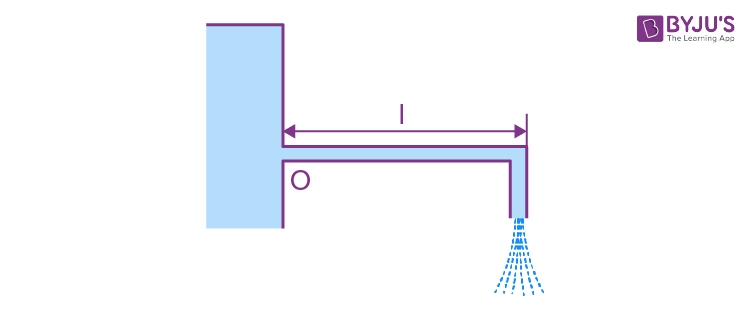Solution:

1. Let the velocity of water flowing through the tube at a certain instant of time be u, then

u = Q/πr2, where Q is the rate of flow of water and πr2 is the cross section area of the tube.

From impulse momentum theorem, for the stream of water striking the tube comer, in x

direction in the time interval dt,

Fx dt = -ρQ u dt

or Fx = -ρQu

And similarly Fy = ρQu

Therefore, the force exerted on the water stream by the tube,

\vec{F}=-\rho Qu\vec{i}+\rho Qu\vec{j}

According to third law, the reaction force on the tube’s wall by the stream equals

-\vec{F}=\rho Qu\vec{i}-\rho Qu\vec{j}

Hence the sought moment of force about 0 becomes

\left | \vec{N} \right |=\frac{\rho Q^{2}l}{\pi r^{2}}

= 0.70 Nm.

15. A side wall of a wide open tank is provided with a narrowing tube (Fig. 1.88) through which water flows out. The cross sectional area of the tube decreases from S = 3.0 cm2 to s = 1.0 cm2. The water level in the tank is h = 4.6 m higher than that in the tube. Neglecting the viscosity of the water, find the horizontal component of the force tending to pull the tube out of the tank.Solution:

1. Suppose the radius at A is R and and it decreases uniformly to r at B where S = πR2 and s = πr2. Assume also that the semi vertical angle at 0 is α. Then

R/L2 = r/L1 = y/x

So y = r + (R - r)(x - L1)/(L2 - L1)

where y is the radius at the point P distant x from the vertex O. Suppose the velocity with which the liquid flows out is V at A, v at B and u at P. Then by the equation of continuity

πr2V = πr2v = πy2u

The velocity v of efflux is given by

v = √(2gh)

And Bernoulli’s theorem gives

pp + ½ ρu2 = p0 + ½ ρv2

where pp is the pressure at P and p0 is the atmospheric pressure which is the pressure just outside of B. The force on the nozzle tending to pull it out is then

F = \int (p_{p}-p_{0})\sin \theta 2\pi yds

We have subtracted p0 which is the force due to atmospheric pressure the factor sin θ gives horizontal component of the force and ds is the length of the element of nozzle surface,

ds = dx sec θ

And tan θ = (R - r)/(L2 - L1)

Thus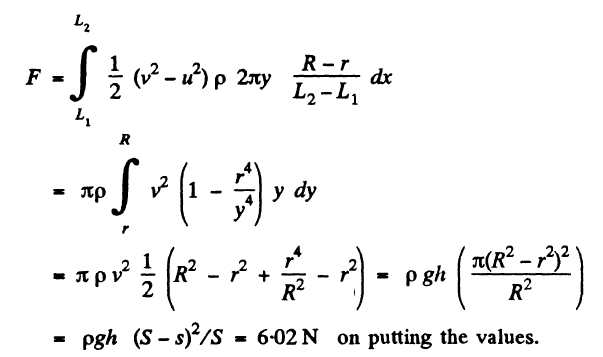Note : If we try to calculate F from the momentum change of the liquid flowing out will be wrong even as regards the sign of the force. There is of course the effect of pressure at S and s but quantitative derivation of F from Newton's law is difficult.

16. A cylindrical vessel with water is rotated about its vertical axis with a constant angular velocity ω. Find:

1. (a) the shape of the free surface of the water;

2. (b) the water pressure distribution over the bottom of the vessel along its radius provided the pressure at the central point is equal to P0.

Solution:

1. The Euler’s equation is

\rho \frac{d\vec{v}}{dt}=\vec{f}-\vec{\triangledown} p

in the space fixed frame where

\vec{f}=-(\rho g\vec{k}) downward.

We assume incompressible fluid so ρ is constant.

Then \vec{f}=-\vec{\bigtriangledown }(\rho gz)

where z is the height vertically upwards from some fixed origin. We go to rotating frame where the equation becomes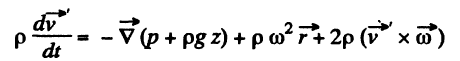The additional terms on the right are the well known coriolis and centrifugal forces. In the frame rotating with the liquid \vec{v}=0

\vec{\bigtriangledown }(p+\rho gz-\frac{1}{2}\rho \omega ^{2}r^{2})=0

p + ρgz - ½ ρω2r2 = constant

On the free surface p = constant, thus

z = ω2r2/2g + constant

If we choose the origin at point r = 0 (i.e. the axis) of the free surface then “constant” = 0 and

z = ω2r2/2g (the paraboloid of revolution)

At the bottom z = constant

So p = ½ ρω2r2 + constant

If p = p0 on the axis at the bottom, then

p = p0 + ½ ρω2r2

17. A thin horizontal disc of radius R = 10 cm is located within a cylindrical cavity filled with oil whose viscosity η = 0.08 P (Fig. 1.89). The clearance between the disc and the horizontal planes of the cavity is equal to h = 1.0 mm. Find the power developed by the viscous forces acting on the disc when it rotates with the angular velocity ω = 60 rad/s. The end effects are to be neglected.Solution:

1. When the disc rotates the fluid in contact with, corotates but the fluid in contact with the walls of the cavity does not rotate. A velocity gradient is then set up leading to viscous forces. At a distance r from the axis the linear velocity is ω r so there is a velocity gradient ωr/h both in the upper and lower clearance. The corresponding force on the element whose radial width is dr is

(η2πr dr)ωr/h (from the formula F = ηA dv/dx)

The torque due to this force is η2πr dr (ωr/h)r

And the net torque considering both the upper and lower clearance is

2\int_{0}^{R}\eta 2\pi r^{3}dr\frac{\omega }{h}

= πR4ωη/h

So power developed is

P = πR4ω2η/h = 9.05 W (on putting the values)

(As instructed end effects i.e. rotation of fluid in the clearance r > R has been neglected.)

18. A long cylinder of radius R1 is displaced along its axis with a constant velocity v0 inside a stationary coaxial cylinder of radius R2. The space between the cylinders is filled with viscous liquid. Find the velocity of the liquid as a function of the distance r from the axis of the cylinders. The flow is laminar.

Solution:

1. Let us consider a coaxial cylinder of radius r and thickness dr. then force of friction or viscous force on this elemental layer, F = 2πrlη dv/dr

This force must be constant from layer to layer so that steady motion may be possible.

Or F dr/r = 2πlη dv (1)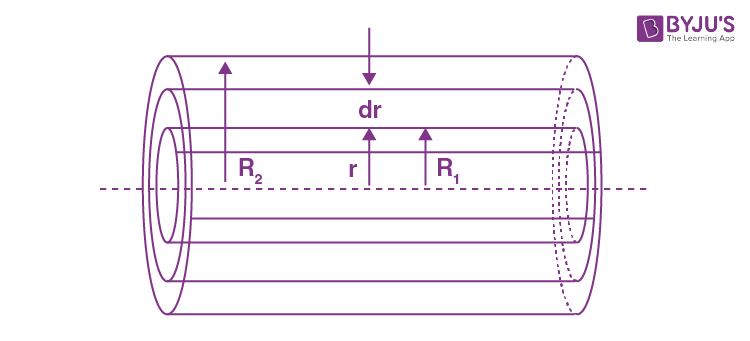Integrating,

F\int_{R_{2}}^{r}\frac{dr}{r}=2\pi l\eta \int_{0}^{v}dv

Or F ln(r/R2) = 2πlηv (2)

Putting r = R1, we get

F ln (R1/R2) = 2πlηv0

From (2) by (3) we get

v = v0 ln (r/R2)/ln(R1/R2)

Note: . The force F is supplied by the agency which tries to carry the inner cylinder with velocity v0.

19. A fluid with viscosity η fills the space between two long co-axial cylinders of radii R1 and R2 , with R1 < R2 axial cylinders . The inner cylinder is stationary while the outer one is rotated with a constant angular velocity ω2 . The fluid flow is laminar. Taking into account that the friction force acting on a unit area of a cylindrical surface of radius r is defined by the formula σ = ηr(∂ω/∂r),

find:

1. (a) the angular velocity of the rotating fluid as a function of radius r;

2. (b) the moment of the friction forces acting on a unit length of the outer cylinder.

Solution:

1. (a) Let us consider an elemental cylinder of radius r and thickness dr then from Newton’s formula

F = 2πrlηr dω/dr = 2πlηr2dω/dr (1)

And moment of this force acting on the element,

N = 2πr2lη (dω/dr)r

= 2πr3lη (dω/dr)

Or 2πlη dω = N dr/r3 (2)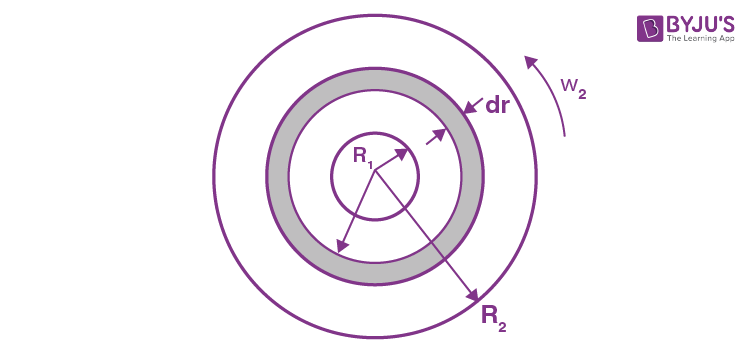As in the previous problem N is constant when conditions are steady.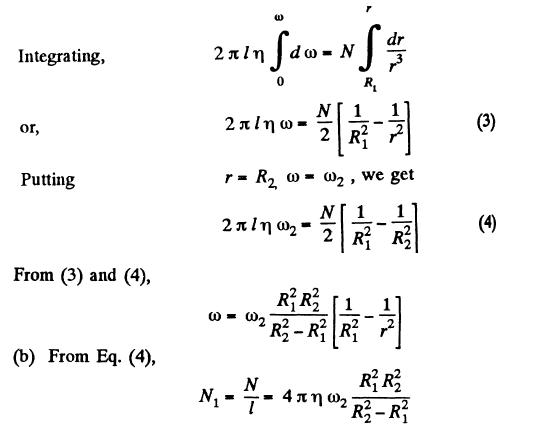20. In the arrangement shown in Fig. 1.90 a viscous liquid whose density is ρ = 1.0 g/cm3 flows along a tube out of a wide tank A. Find the velocity of the liquid flow, if h1 = 10 cm, h2 = 20 cm, and h3 = 35 cm. All the distances l are equal.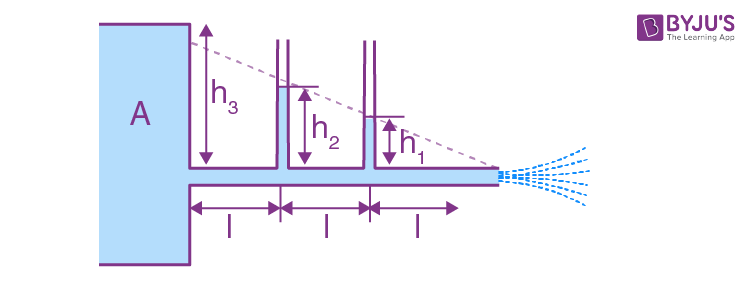Solution:

1. The loss of pressure head in travelling a distance l is seen from the middle section to be h2 - h1 = 10 cm. Since h2 - h1 = h1 in our problem and h3 - h2 = 15 cm = 5 + h2 - h1, we see that a pressure head of 5 cm remains uncompensated and must be converted into kinetic energy, the liquid flowing out. Thus

ρv2/2 = ρg∆h where ∆h = h3 - h2

Thus v = √(2g∆h) = 1 m/s